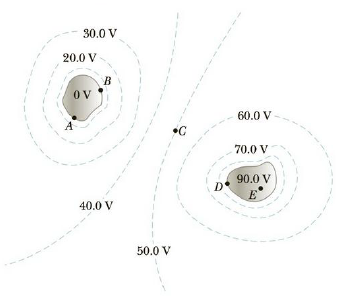Chapter 16, Problem 3CQ

Chapter
Section
Textbook Problem

Figure CQ16.3 shows equipotential contours in the region of space surrounding two charged conductors. Find (a) the work WAB in electron volts done by the electric force on a proton that moves from point A to point B. Similarly, find (b) WAC, (c) WAD, and (d) WAE.Figure CQ16.3

(a)

To determine
The work done WAB .

Explanation

Formula to calculate the work done WAB is,

WAB=q(VBVA)

• q is the charge.
• VA is the potential at the point A.
• VB is the potential at the point B.

Substitute e for q, 0 V for VA and 0 V for VB

(b)

To determine
The work done WAC .

(c)

To determine
The work done WAD .

(d)

To determine
The work done WAE .

Still sussing out bartleby?

Check out a sample textbook solution.

See a sample solution

The Solution to Your Study Problems

Bartleby provides explanations to thousands of textbook problems written by our experts, many with advanced degrees!

Get Started